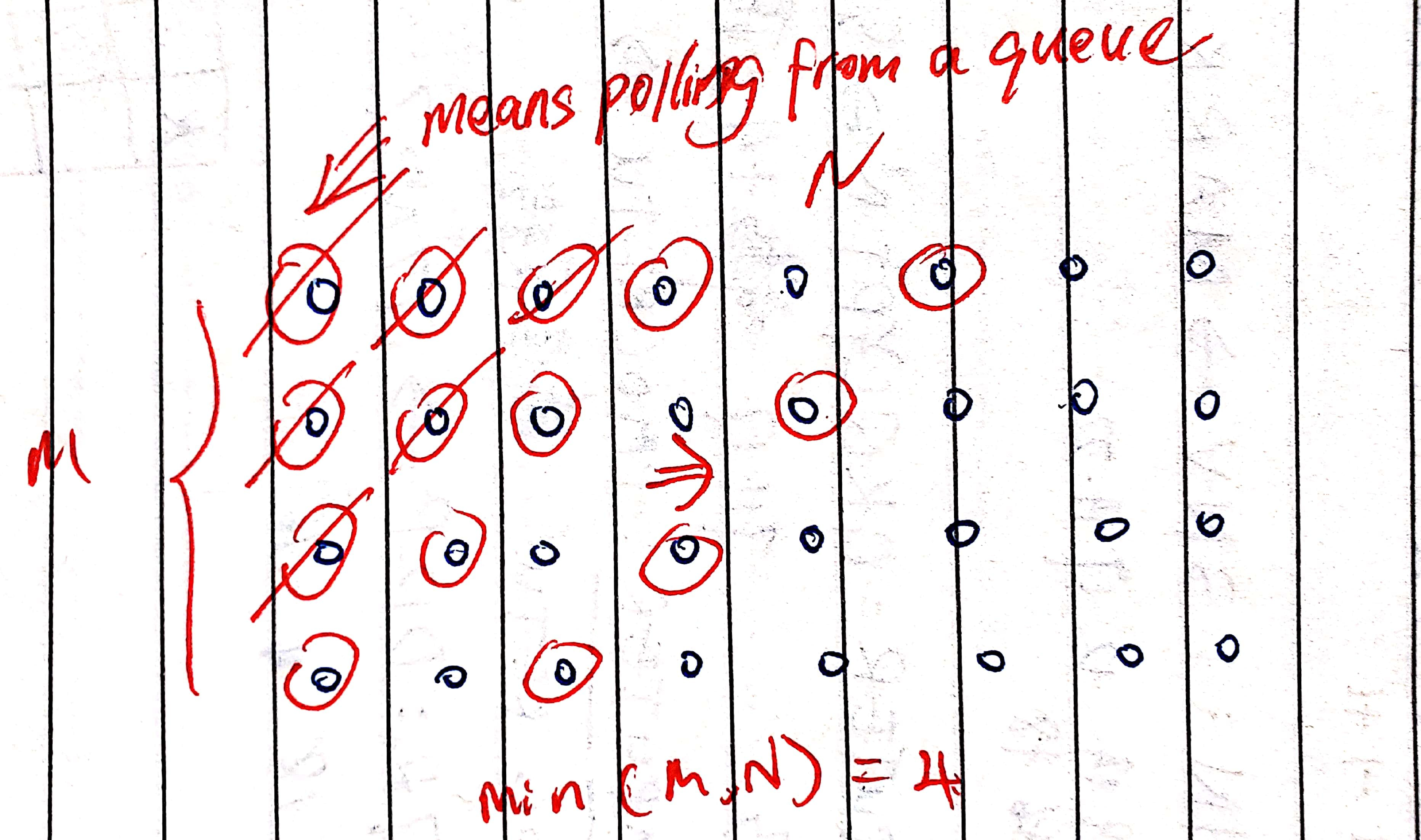# 200. Number of Islands

Reference: LeetCode
Difficulty: Medium

## Problem

Given a 2d grid map of '1's (land) and '0's (water), count the number of islands. An island is surrounded by water and is formed by connecting adjacent lands horizontally or vertically. You may assume all four edges of the grid are all surrounded by water.

Example:

## Analysis

### DFS

It is very obvious that this problem can be solved by using an DFS approach.

Note: Be careful of the conditions that we dfs a place, which include boundary check and whether it is an island.

Time: $O(MN)$
Space: $O(MN)$. If we modify the original grid array, can we reduce the complexity to $O(1)$? No. It is because DFS goes by a call stack.

### BFS

A similar idea based on BFS using a queue.

Time: $O(MN)$
Space: $O(\min{(M, N)})$. In the worst case where the grid is filled with lands, the size of queue can be $O(\min{(M, N)})$ as follows.CommentJunhao Wang
a software engineering cat